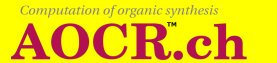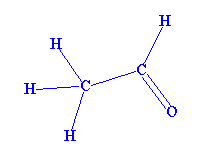# 1. Organic chemistry basics

This chapter provides you with the basic concepts needed to understand how the program operates. These basic concepts are organic compounds, reactions and mechanisms.

### 1.1. Formulas

Figure 1.1A group of organic compounds (organic molecules) is represented by a formula where symbols like H, O, N, C, ..., are possibly connected by single, double, triple, or quadruple bonds and are possibly carrying a number of positive or negative charges and of electrons, that appear for example in radicals. The formula of the compound acetaldehyde can be seen in Figure 1.1.

Subsection 3.1.1 explains how to build a formula with the Graphic Composer.